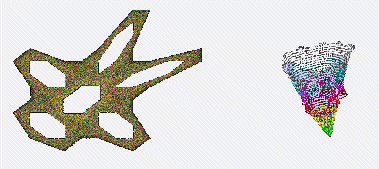### Simple Iterations

Here we look at a second example. The third one is presented in the partition Iterations II.

Kaneko Attractor Machine

a: b: scale:

Typ I Typ II

Use the Kaneko machine by changing the values in the edit fields at the bottom of the drawing canvas. Press the draw button and watch the new figure. The table below shows sample values. If the figure is too big, decrease the scale factor. The radio checkboxes below choose the Kaneko square type I or the linear type II. The color is changed every 1000 dots, until the 10 predefined colors are used, then the colors are repeated.

The FormulaA

B

Scale

Type

0.1

1.67

160

I

0.5

4

180

I

0.17

1.3

200

II

0.562

2.76

250

II

Hint: Choose A, B > 0

Javascript codes for three machines

The code for controlling is integrated in the source code of this HTML page. The source code for the Mira, Kaneko and Martin machine you'll find in the embedded file iterations.js.Other Orbit-Fractals

There are more similar fractals, p.e. the Gingerbreadman with a very simple iteration formula:

xn+1 = 1 - yn + |xn|
yn+1 = xn

One more example is a three-dimensional-looking fractal, although being calculated in two dimensions is the Kamtor3D.The iteration formula depends on angle functions:

xn+1 = xn * cos a + (xn* xn - yn) * sin a
yn+1 = xn * sin a - (xn * xn - yn) * cos a